Divisible

Chapter 4 Class 11 Mathematical Induction
Concept wise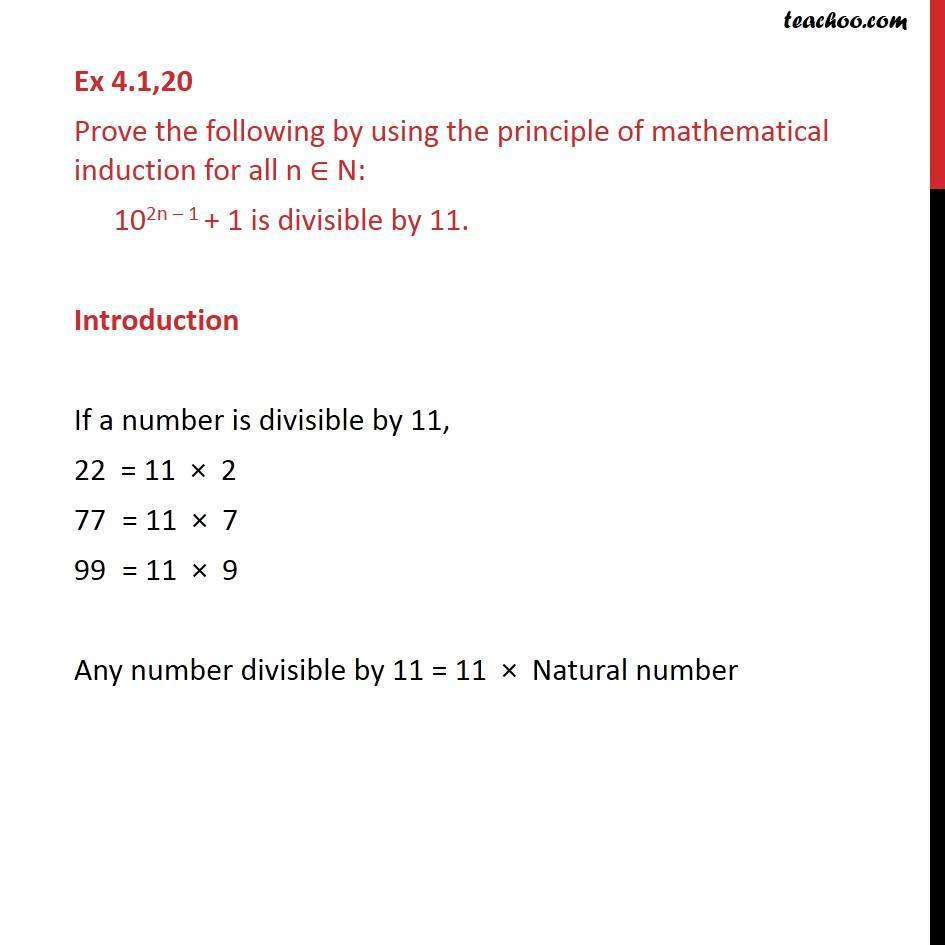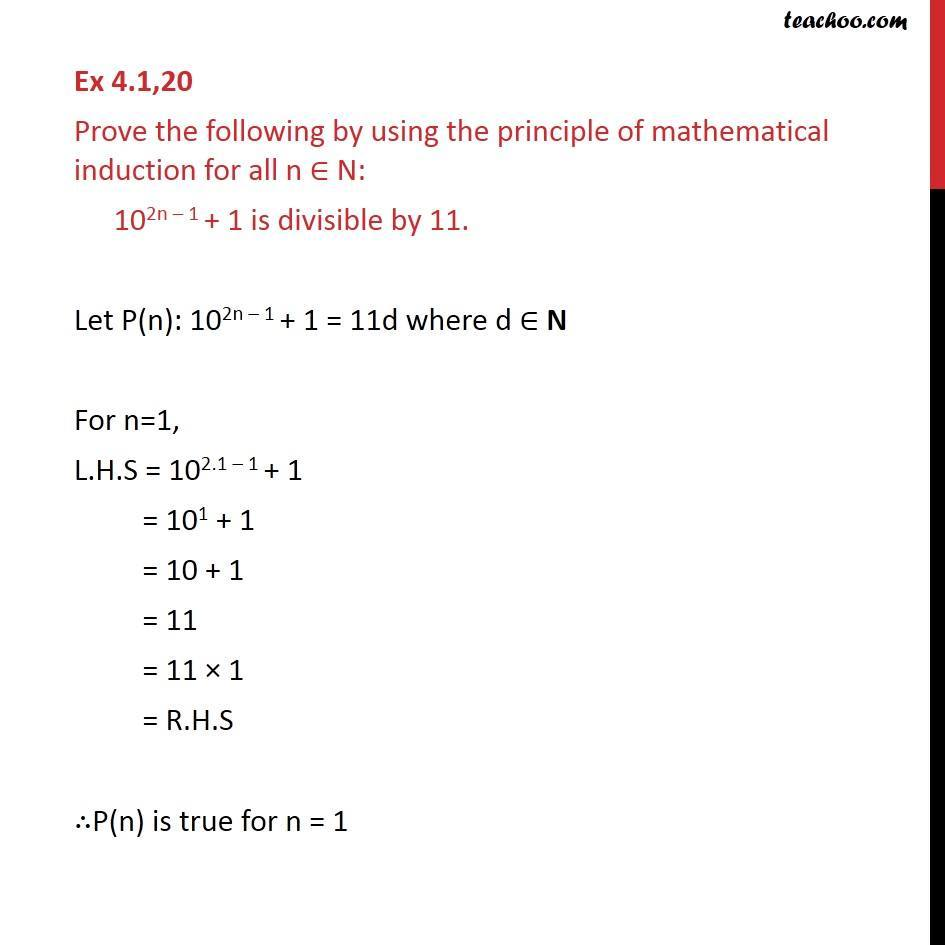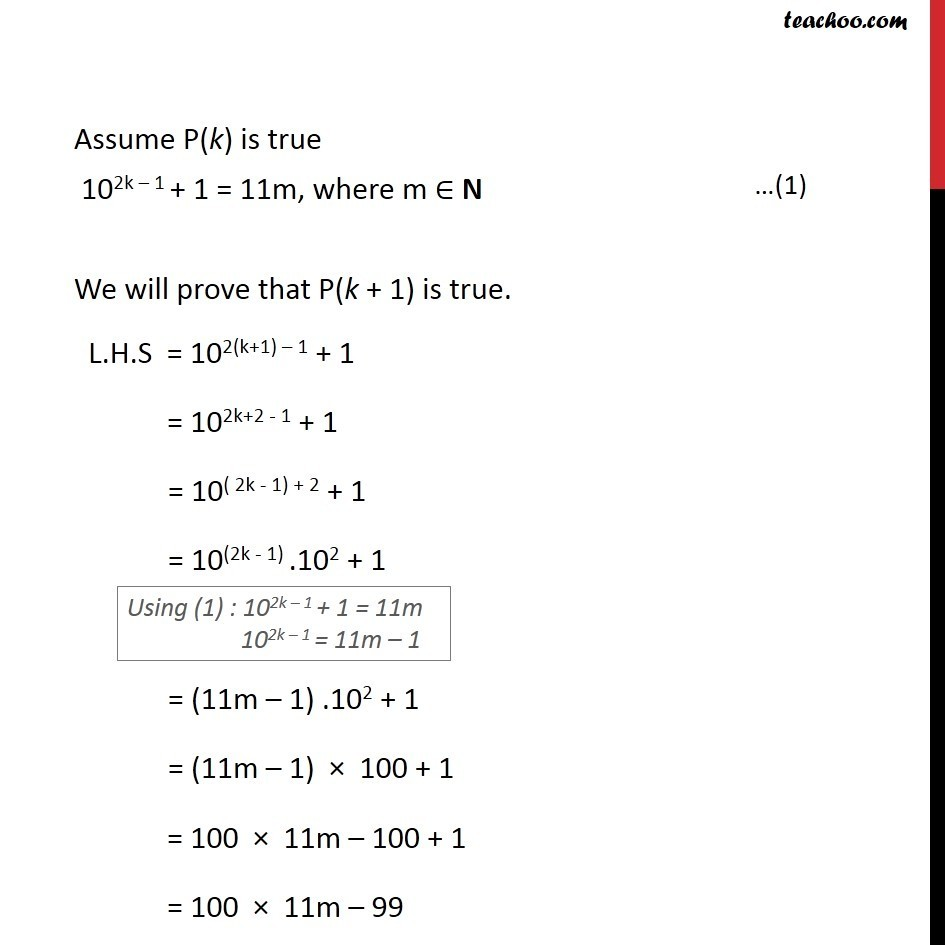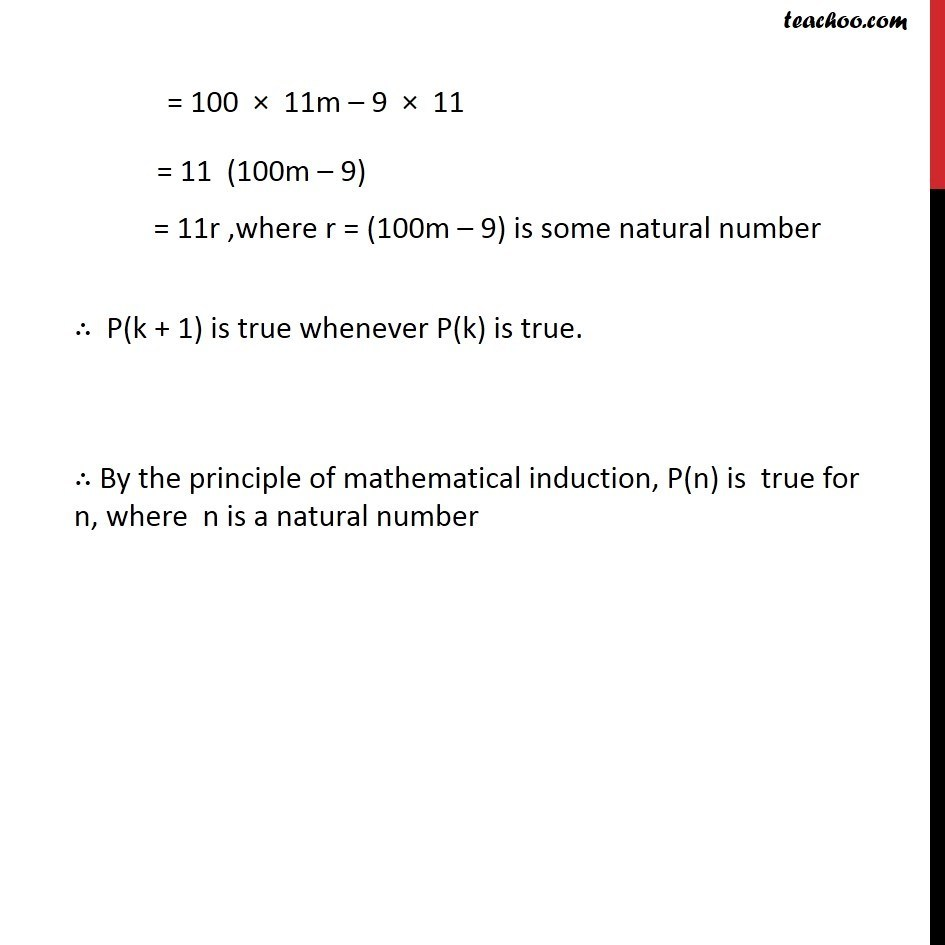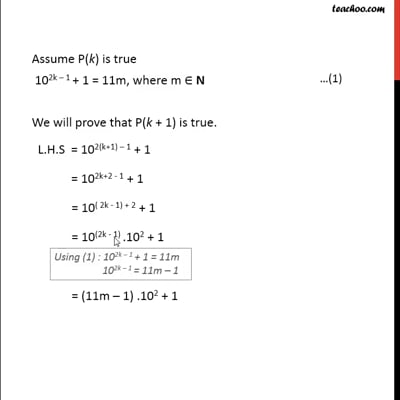This video is only available for Teachoo black users

Introducing your new favourite teacher - Teachoo Black, at only ₹83 per month

### Transcript

Ex 4.1,20 Prove the following by using the principle of mathematical induction for all n ∈ N: 102n – 1 + 1 is divisible by 11. Introduction If a number is divisible by 11, 22 = 11 × 2 = 11 × 7 = 11 × 9 Any number divisible by 11 = 11 × Natural number Ex 4.1,20 Prove the following by using the principle of mathematical induction for all n ∈ N: 102n – 1 + 1 is divisible by 11. Let P(n): 102n – 1 + 1 = 11d where d ∈ N For n=1, L.H.S = 102.1 – 1 + 1 = 101 + 1 = 10 + 1 = 11 = 11 × 1 = R.H.S ∴P(n) is true for n = 1 Assume P(k) is true 102k – 1 + 1 = 11m, where m ∈ N We will prove that P(k + 1) is true. L.H.S = 102(k+1) – 1 + 1 = 102k+2 - 1 + 1 = 10( 2k - 1) + 2 + 1 = 10(2k - 1) .102 + 1 = (11m – 1) .102 + 1 = (11m – 1) × 100 + 1 = 100 × 11m – 100 + 1 = 100 × 11m – 99 = 100 × 11m – 9 × 11 = 11 (100m – 9) = 11r ,where r = (100m – 9) is some natural number ∴ P(k + 1) is true whenever P(k) is true. ∴ By the principle of mathematical induction, P(n) is true for n, where n is a natural number